# 2nd PUC Basic Maths Question Bank Chapter 21 Definite Integral and its Applications to Areas Ex 21.1

Students can Download Basic Maths Exercise 21.1 Questions and Answers, Notes Pdf, 2nd PUC Basic Maths Question Bank with Answers helps you to revise the complete Karnataka State Board Syllabus and score more marks in your examinations.

## Karnataka 2nd PUC Basic Maths Question Bank Chapter 21 Definite Integral and its Applications to Areas Ex 21.1

Part-A

2nd PUC Basic Maths Definite Integral and its Applications to Areas Ex 21.1 One Mark Questions and Answers

Question 1.
$$\int_{0}^{1} x^{2} d x$$
$$\int_{0}^{1} x^{2} d x=\left[\frac{x^{3}}{3}\right]_{0}^{1}=\frac{1}{3}$$

Question 2.
$$\int_{1}^{2}\left(x+e^{x}\right) d x$$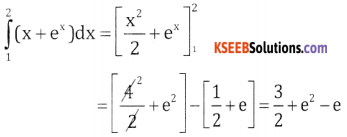Question 3.
$$\int_{0}^{\frac{\pi}{2}} \sin 2 x d x$$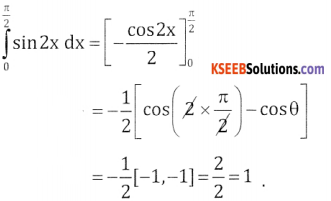Question 4.
$$\int_{\frac{-\pi}{4}}^{\frac{\pi}{4}} \csc ^{2} x d x$$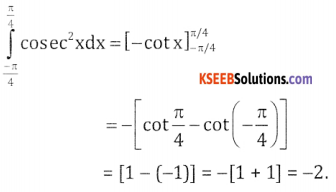Part-B

2nd PUC Basic Maths Definite Integral and its Applications to Areas Ex 21.1 Three and Five Marks Questions and Answers

Question 1.
$$\int_{1}^{2} \frac{2 x+5}{\left(x^{2}+5 x+3\right)^{2}} d x$$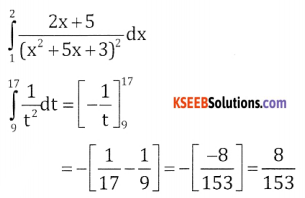put x2 + 5x + 3
(2x + 5) dx = dt
If x = 1, t = 1 + 5 + 3 = 9
If x = 2, t = 4 + 10 + 3 = 17Question 2.
$$\int_{-1}^{1}(10 x+3) \sqrt{5 x^{2}+3 x+7} \cdot d x$$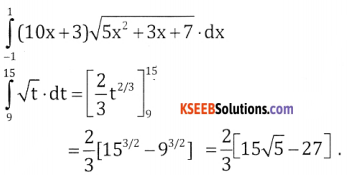Question 3.
$$\int_{0}^{1} \frac{2 x+3}{3 x+5} d x$$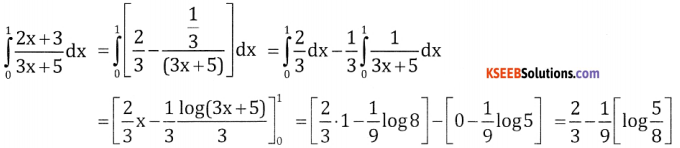Question 4.
$$\int_{0}^{\frac{\pi}{2}} x \sin x d x$$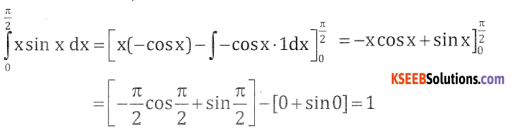Question 5.
$$\int_{2}^{3} \frac{1}{(x+1)(x+2)} d x$$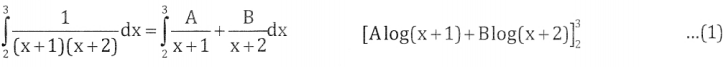∴ $$\int_{2}^{3} \frac{1 \cdot d x}{(x+1)(x+2)}$$ = [1. log(3 + 1) – 1 log (3 + 2)] – [log(2 + 1) – log(2 + 2)]
= 2 log 4 – log (5 × 3) = log 42 – log 15 = log ($$\frac { 16 }{ 15 }$$)